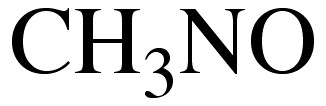## Combichem: an introductory example of the complexity of chemistry

Chemistry gets complex very rapidly. Consider the formula CH3NO as the topic for a tutorial in introductory chemistry. I challenge my group (of about 8 students) to draw as many different molecules as they can using exactly those atoms. I imply that perhaps each of them might find a different structure; this normally brings disbelieving expressions to their faces.Click on image to see molecules constructed from these atoms. The list is not comprehensive!

Amongst the useful concepts that can be introduced are:

1. How to determine how many double bond equivalents (or degrees of unsaturation) are implied by the formula.
1. Students spot one dbe in the above formula, but can take a little longer to notice that it can reside in a ring.
2. Few (and I count tutors in this) will add sub-valent atoms (here, the possibility of a carbene or a nitrene) to the list.
2. What is meant by “different”? This can be reduced to the equations: Ln k/T = 23.76 – ΔG/RT; t1/2 = (Ln 2)/k, where t1/2 is the half life (in seconds) of any species constrained by a free energy barrier of ΔG. A nice illustration of this equation is to be found on Jan Jensen’s blog (and an worthwhile calculation would be to find the barrier required to achieve a half life based on the age of the universe). This can be boiled down to three ranges.
1. Half lives of ~10-15 s, or vibrations (and this includes transition states themselves). Arguably, resonance isomers, which involve the (nominal) motions of electrons and not nuclei, fall into this class as well.
2. Half lives of < 101 s, which would include most conformational isomers (excepting atropisomers) and highly unstable isomers, and which cannot be bottled and labelled as such.
3. Compounds with half lives > 102 s, up to of course the age of the universe. This would include configurational isomers (and if the students are up to it, you can ask them to identify any compounds constructed above which can exhibit optical isomerism).
3. One might be inclined to (approximately) use arrows to indicate the timescales above. Thus electronic resonance is represented by double-headed arrow, conformational and E/Z isomers by an equilibrium arrow, and a single headed arrow indicating a reaction (which may in fact have a very low barrier) connecting two isomers.
4. Its normally now time to count the electrons. This includes the “invisible ones”, the lone pairs, and also the occasion to introduce the valence shell octet.
5. Putting the appropriate charges onto any atoms which require them is always fun (the dative bond is avoided). The blue structure revealed in the click above is an extreme interpretation of this! Gernot Frenking has pioneered the class of compound he calls carbones. For his latest article on the theme, see DOI: 10.1002/anie.201002773. The green compound would belong to this class, if it did not fall apart (probably with no barrier) to something which is not actually one molecule, but two (separable) molecules (purple). This brings us into what a molecule actually is. Could it be two molecules unconected by any bonds, but nevertheless also inseparable (such as catenanes, rotaxanes, and many other entwined systems)? Two molecules can also interact weakly, which is not normally referred to as bonds. In this case, the two molecules would be bound by a hydrogen bond.
6. Quite a number of the isomers can be also called tautomers. This involve the movement of one type of atom in particular, the hydrogen (or proton). In terms of lifetime, they would fall into class 2 above (although if one takes extreme care to remove all traces of acids or bases, particularly from the surface of any glass container, one can extend the lifetimes quite considerably).
7. The peptide bond is included in the isomers, and its ionic resonance formulation, which can lead the discussion to the molecular basis of life and how finely-tuned this bond in fact is.
8. One might speculate about what the most stable of all the isomers might be, and how many are indeed bottleable. One might introduce quantum mechanics as nowadays a very reliable way of estimating this (and whilst you are at it, introduce free energies, entropies etc). For example, which of the two red geometrical isomers is the more stable, and why? What is the best resonance representation (i.e. where does one put the charges? On this specific point, a CCSD/6-311G(d,p) ELF calculation does come up with a very definitive answer of on the nitrogen rather than the oxygen).
9. This might be followed up by introducing arrow pushing as a means of interconverting two isomers, and with one of the pair of isomers, one can introduce pericyclic selection rules, transition state aromaticity and other advanced stereochemical concepts.
10. Now we are well into to stereoelectronics. One can introduce anomeric effects via the NBO technique. Thus in the red compounds, there is an interesting interaction between the lone pair on carbon and the anti N-H bond (but, spectacularly, not the syn N-H bond). There is another particularly strong one between the oxygen lone pair and the C-N bond.

I dare say I have only picked at the surface, but covering the above should be enough for one tutorial I should imagine 🙂

PS For the (calculated) relative energies of some of these isomers, see DOI: 10.1021/jo010671v